# International Short Date Formatting for the TEXT Function

I have an Excel workbook that is used in two different regions where the date format is entirely different, the US and the UK. At the top of a report worksheet I use a TEXT function to inform the user of the date range.

Here’s what a US user sees:

From: 6/6/2011 to 6/10/2011

Here is the formula:

=”From: ” & TEXT(MIN(ExtractData!A:A),”m/d/yyyy”) & ” to ” & TEXT(MAX(ExtractData!A:A),”m/d/yyyy”)

The Min and Max dates are in column A on a worksheet named ExtractData.

The problem is trying to automatically change the date format in the second argument of the TEXT Function – “m/d/yyyy” – which is a string argument. We can use VBA to accomplish this, but first a refresher on the TEXT function syntax.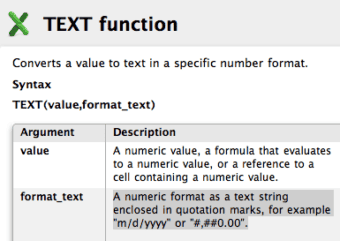## The Solution

The Application.International Property solves this problem with the xlMDY argument, which is TRUE if the date order is month-day-year, and FALSE if the date order is day-month-year. This property is put into the Workbook_Open event and modifies a defined name constant that’s used for the second argument of the TEXT function.

Here’s how it’s done.

## Create a Defined Name Constant for the Date Format

I created a Defined Name Constant to store a Short Date format, and gave it the name sd_format.

In Excel 2007 and 2010, choose Formulas > Define Name to bring up the New Name dialog box where you type in sd_format in the Name box, and type =”m/d/yyyy” in the Refers to box. Remember the equals sign.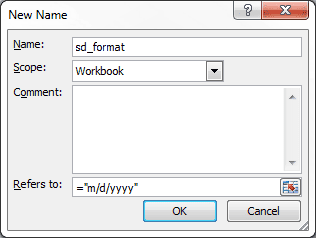In Excel 2003, 2008, and 2011 choose Insert > Name > Define to bring up the Define Name dialog box. Type sd_format in the Names in workbook box, then type =”m/d/yyyy” in the Refers to box. Be sure to use the equals sign.

## Substitute the Named Constant in the TEXT Function

The sd_format defined name can now be substituted for “m/d/yyy” in the second argument of the TEXT function. Here’s the new formula:

=”From: ” & TEXT(MIN(ExtractData!A:A),sd_format) & ” to ” & TEXT(MAX(ExtractData!A:A),sd_format)

Since sd_format is already a text string, enclosed quotes are not needed.

## Create a Workbook Open Routine

In the VBA Editor, I created a Workbook_Open subroutine, which looks at the computers international setting for the US, and if TRUE changes the sd_format value to “m/d/yyy”, and otherwise changes it to “d/m/yyyy” for the UK short date format.
``` Private Sub Workbook_Open() '''''''''''''''''''''''''''''''''''''''''''''''''''''''''''''''''' ' This routing updates the regional date setting format for the ' defined name sd_format. ''''''''''''''''''''''''''''''''''''''''''''''''''''''''''''''' If Application.International(xlMDY) = True Then ThisWorkbook.Names("sd_format").Value = "m/d/yyyy" Else ThisWorkbook.Names("sd_format").Value = "d/m/yyyy" End If End Sub ```

## How it All Works

Each time the workbook is opened the Workbook_Open routine executes the IF-THEN-ELSE statement, which simply looks to see if the computers region setting is month-day-year, then sets the defined name sd_format to “m/d/yyyy” which is a US format. If the computer’s region setting for the long date format is NOT month-day-year (and presumably day-month-year) then sd_format is set to “d/m/yyyy” for the UK.

Every TEXT function using sd_format for the second argument will then have the proper short date format for that computer.

Note: Obviously if the region settings are changed on the computer while the file is open the file will have to be closed and reopened, but this would most likely be a rare occurrence.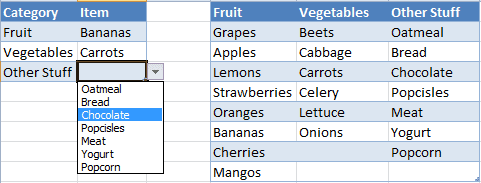# A Dynamic Dependent Drop Down List in Excel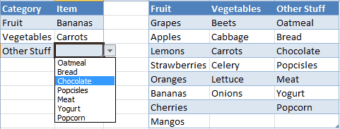The other day I was reading a post over at the Contextures blog about Dynamic Dependent Excel Drop Downs and realized that using an Excel Table would provide an alternative method that is both simple and flexible. Tables are available in Excel versions 2007, 2010, and 2011.

In this post I’ll create a Table to hold the Category’s and Items, create three defined names using dynamic formulas, then use Data Validation to create two drop-down lists, the second being dependent upon the first.

## Create at Reference Table

Here’s a Table with Category names in the header row and Items in the columns. I just typed in the information then converted to a Table.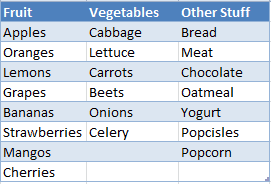## Create a Dynamic Defined Name for Category List

Create a defined name for the Table1 Header row range by using the formula =Table1[#Headers]. Please note that Table1 is the name of the Table created in the step above.

This defined name is dynamic, meaning it will expand when more columns are added and shrink if any columns are deleted. It will return the header row of the Table, which we’ll use in the next step. I used myCategory for this defined name.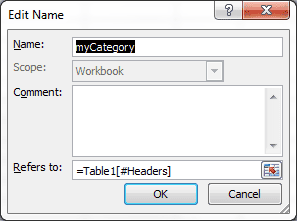To create a defined name in Windows choose Formulas > Name Manager then click New…. On a Mac choose Insert > Name > Define…. This will bring up the New Name dialog box that looks like the Edit Name screen-shot shown above.

## Create a Category Drop Down List with Data Validation

Type Category in cell A1 for the column heading. Next select cell A2, then choose Data > Data Validation > Data Validation… and in the Data Validation dialog box (shown below) select List from the Allow box, then type in =MyCategory in the Source box, and click OK.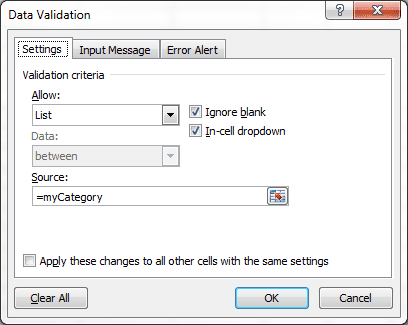Now cell A2 has a drop down button that shows the Header row of the Table. (Don’t worry about extending this Data Validation down to more rows, we’ll take care of that later.)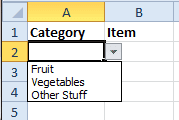Column B will hold a drop-down list for the Item, which is dependent upon the Category. This takes two defined names to work properly.

## Create the First Defined Name for Items

[Update: Select cell B2 before you follow this next step.]
Create a defined name with the following formula:

that I’ll name myItemList.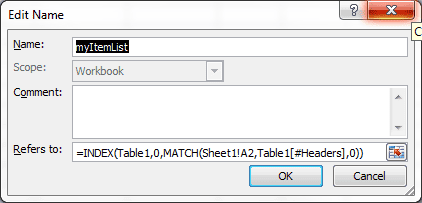This formula will return a reference to the Table column that matches the Category selection in cell A2 on Sheet1.

If I use Data Validation to create a drop-down list with the myItemList defined name I’ll get eight items returned because Table1 has eight data rows. As you can see in the picture below, the Vegetables item list has two blank lines, and the Other Stuff item list has one blank line.  Not an elegant solution.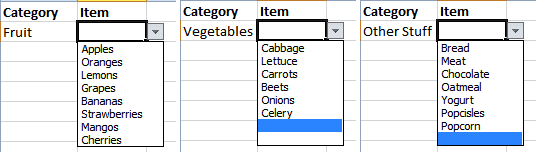However, if you have a table that always has equal items (rows) in each column then this defined name formula will will work well for a Data Validation list.

## Create a Second Defined Name for Items

We can create a second defined name that will give us a dynamic list with the exact count. The following OFFSET formula will do the trick.

=OFFSET(myItemList,0,0,COUNTA(myItemList),1)

This formula uses the first defined name (myItemList) but alters the height by counting the items. I named this defined name myItem, as you can see below in the Name Manager screen shot.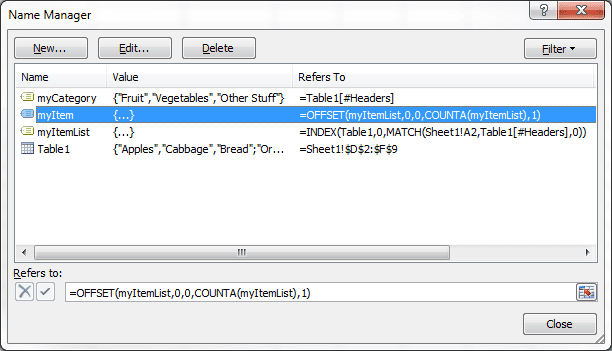## Create an Item Drop Down List with Data Validation

Type Item in cell B1. Select cell B2 and open the Data Validation dialog box. Choose List and enter the following formula =myItem, then click OK.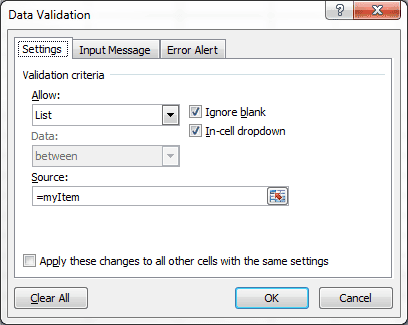The result is a dynamic drop-down list in the Item column that’s dependent on the Category selection in column A, and returns the exact list.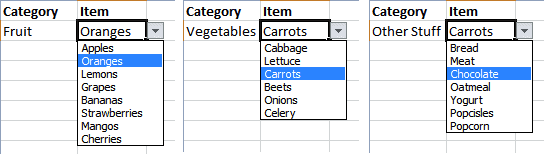## Convert to a Table

Now it’s time to covert this to a Table, and by doing so the Data Validation will be preserved and automatically expand with the addition of more rows.

Select cell A2, then in Windows (Excel 2007, 2010) choose Insert > Table, verify the information in the Create Table dialog box, and click OK.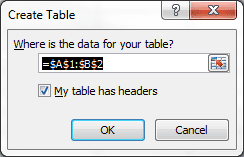On a Mac (Excel 2011), select cell A2 then choose Tables > New > Insert Table with Headers.

At this point I normally turn off the Data Filter because it’s rather annoying.

As your Table expands, with more rows or columns, this dynamic drop-down list will work just fine. To create a new row in the Table with Data Validation press the Tab key while the active cell is the last column of the last row.

### Potential Problem with OFFSET

As you might have guessed the OFFSET formula depends on having items at the top of the list. Should your data have blank rows in the middle of the column, the drop-down won’t have all the items listed yet show blank cells in the list.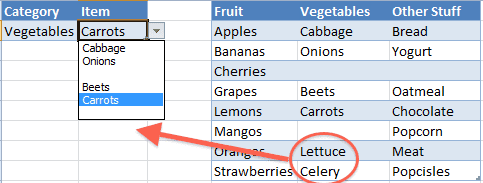In this case it’s best to use the first defined name (myItemList) in creating a dependent, drop-down list for Item. At least you’ll get all the data, even if it does have some blanks in the list.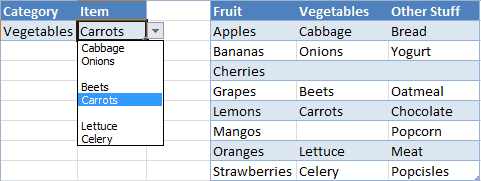## Reference Table Location

For illustration purposes I’ve shown the reference Table on the same worksheet as the Category and Item Table. Normally I would place the reference Table on a different worksheet. In this instance none of the formulas would change.

# Convert Coordinates for Your GPS with Excel

A few years ago I found myself just south of Paris, France one Sunday with a car and the inclination to do some sight-seeing. Since I was alone and don’t speak French my saving grace was Google Earth and a Tom Tom GPS navigation device.

Tom Tom allows GPS coordinates to be entered as a destination, but Google Earth lists those coordinates in a different format. In researching this article I found out that GPS coordinates can be presented in at least four different formats, making it difficult to understand the coordinates.

### Google Maps view of the Eiffel Tower

Click the x to remove the Address box and show the interactive Google Map.

## Use Excel to Convert GPS Coordinates for Tom Tom

One of my destinations was to see the Eiffel Tower and Google Earth shows the GPS coordinates to be 48 degrees 51 minutes 32.64 seconds North and 2 degrees 17 minutes 34.90 seconds East. (Coordinate formatting shown as 48° 51′ 29.69″ N 2° 17′ 38.02″ E in Google Earth while holding the mouse over a position on the map.)

However the Tom Tom GPS device wanted coordinate input in degrees only, where minutes and seconds represent the fractional part. So I created a quick spreadsheet to do the conversion. (Click the picture to download the .xls file.)To convert from Degrees, Minutes, Seconds to a Tom Tom degree format, the math is:

Degrees+(Minutes/60+Seconds/3600)

This allowed me to enter GPS coordinates directly into the Tom Tom GPS device. Since I wasn’t familiar with the street names or the numbering system for locating addresses on the Tom Tom, this was much faster than trying to find the correct address in the Tom Tom street directory. The Tom Tom GPS was a loner from an associate so that was another factor in my decision to use GPS coordinates.

Note: Tom Tom allows different formats for GPS coordinates, this example just mirrored settings for the GPS I was using at the time.

## Use Excel to Convert GPS Coordinates for Garmin

When I got back home to the USA and tried this with my Garmin it didn’t work because the required format for GPS coordinates was different. My Garmin GPS device wanted Degrees and Minutes only, with seconds being the fractional part of minutes. That formula is different because it requires some concatenation with an empty space between degrees and minutes.

Degrees & ” ” & Minutes+Seconds/60

So my Excel spreadsheet helped me convert numerous GPS coordinates for my trip to Paris.Note: If you’re wondering about the North and East designations, click this link to see how to read GPS coordinates.

## Convert GPS Coordinates with Excel

Here’s an interactive worksheet that you can use to figure out some GPS coordinates for yourself.

Cross-posted at vlatte.net.

# A Woopra Chat About ExcelI use the Woopra desktop app to view live visitors to this WordPress blog. Visitors will see a Woopra window lurking about when I’m logged into my (free) Woopra account. One option is to initiate a chat session with me.

My first chat happened the other day. The chat notice startled me, but I quickly recovered and was able to interact with a visitor.

(Bold and color are my additions for clarity)

Visitor #13357:
08:53:19. HI
You:
08:53:28. Howdy

Visitor #13357:
08:54:22. I WANT TO CONVERT 01:05 = 65 MIN WHAT I DO
08:55:42. IN EXCEL
You:
08:56:58. In cell A1 type 1:05, and in cell B1 enter the formula =A1*24*60 then change the cell format to General and you’ll see the 65.

Visitor #13357:
08:57:42. I NEED IN THIS FORMAT
08:57:46. 65 MIN
You:
08:58:41. Change the formula in B1 to =A1*24*60 & ” MIN”

Visitor #13357:
09:00:21. MY TIME FORMAT IN 01:01 AND IT SHOWS 60.9999999999999 MIN
NOT 61 MIN
You:
09:03:04. Try this =TEXT(A1*24*60,”#”) & ” MIN”

Visitor #13357:
09:04:38. THANKS ITS WORKING
09:04:44. THANKS A LOT
You: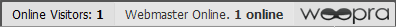The strange thing I just noticed is that some of my replies have periods at the end. In a chat session no less!

# INDEX and MATCH Functions Together Again in Excel

The INDEX and MATCH functions are used together to perform lookups. The INDEX functions grabs a cell from an array, and the MATCH function contributes the row and/or column position.

## INDEX and MATCH Example

Familiarity with the VLOOKUP function can cause you to restrict INDEX and MATCH to less than they can be. The example spreadsheet below bears this out.

The formula for Fiscal QTR takes the Month in cell E2 as input, finds that value in the Month column, then returns the Fiscal QTR in the same row. The first formula is:

=INDEX(A2:B13,MATCH(E2,A2:A13,0),2)

This INDEX formula uses the array range A2:B13 for the first argument, which is what you may use if familiar with VLOOKUP. But this requires that both the second and third arguments are used (row and column position).

The MATCH function looks to cell E2, finds the exact matching value in the Month range A2:A13, and returns the row number 7 to the INDEX Function for it’s second argument. The number 2 is input for the third (column) argument of the INDEX Function. The INDEX function returns the value 1 from row 7, column 2 of the A2:B13 range.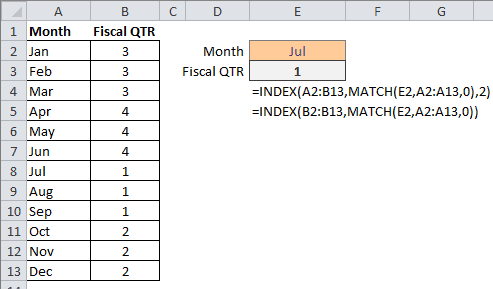The second formula:

=INDEX(B2:B13,MATCH(E2,A2:A13,0))

is different in that INDEX uses a one-column range B2:B13, so only a row number argument is required. The MATCH function looks at Jul in cell E2 and finds a match in range A2:A13, returning the number 7 for the INDEX row number argument.

## A Grade Lookup Example with INDEX and MATCH

Here’s a student grade lookup formula that relies on the match type argument of the MATCH function to be 1, which finds the largest value, less than or equal to the lookup value.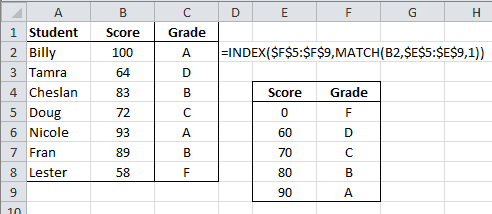The formula in cell C2 is:

=INDEX(\$F\$5:\$F\$9,MATCH(B2,\$E\$5:\$E\$9,1))

The INDEX function uses array range F5:F9 and the MATCH function array range is B2:E9. (The dollar signs (\$) indicate absolute values because I copy the formula down).

The MATCH function looks to cell B2, which has the value 100, then finds an approximate match of 90 in row 5 in the range E5:E9. The 1 indicates this will be an approximate match. The INDEX function then returns the cell in row 5 of the F5:F9 range, which is the text value A.

# The INDEX Function in Excel

The INDEX and MATCH functions working together are more flexible than using the VLOOKUP function. However, if you’ve never used INDEX and MATCH to perform a lookup it can get confusing.

I’m going to cover the INDEX function here so you can learn it well and don’t have to think about it too much when we put it together with the MATCH function.

## The INDEX Function Details

The INDEX function returns a cell value from a range, given a row and/or column position number. The syntax is:

INDEX(array, row_num, [column_num])

• Array is required and is a range of cells or an array constant.
• If array contains only one row or column, the corresponding row_num or column_num is optional.
• If array has more than one row and column, and only row_num or column_num is used, INDEX returns an array of the entire row or column in array.
• Row_num is a row number within the array. If omitted, column_num is required.
• Column_num is a column number within the array. If omitted, row_num is required.

If both row_num and column_num are used, INDEX returns the value in the cell at the intersection of the two within the array.

## Examples of the INDEX Function

In the spreadsheet examples below, array is the range A2:B13, which is also given the named range of fQTR. The first INDEX formula in cell E4 returns the Fiscal Quarter from the array. The formula is =INDEX(A2:B13,E3,2).

The row_num argument is the Month # in cell E3, which is 5. The column_num argument is 2, and entered directly into the formula. The INDEX function looks to the array, pulls out the value from the cell intersecting the the 5th row and 2nd column, and returns the value 4.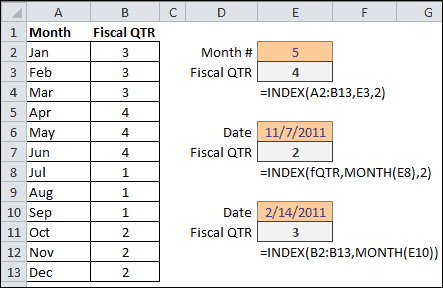The second INDEX function example in cell E9 uses the named range fQTR for the array.  The formula is =INDEX(fQTR,MONTH(E8),2).

The row_number argument uses the MONTH function to pull out the number 11 from the Date in cell E8. The column_num argument is 2 and entered directly in the formula. The INDEX function returns 2 for the Fiscal Quarter, because the 11th month of the Date corresponds to the 11th row and the value in the 2nd column is 2. The value in row 11, column 2, of the fQTR array is 2.

If you wanted to get away from using 2 for the column_num argument, an alternative would be to use COLUMNS(fQTR) or COLUMNS(A2:B13), which would give you the total number of columns or last column in the array range that is 2 in this case. The formula would be =INDEX(fQTR,MONTH(E8),COLUMNS(fQTR)).

In the third example, since each value of Month corresponds to the row number, Jan =1, Feb =2, etc., the INDEX array argument only has to contain the column of data for Fiscal QTR and no column argument is required. The INDEX formula for converting a date to Fiscal QTR is =INDEX(B2:B13,MONTH(E10)).

# The MATCH Function in Excel

The MATCH and INDEX functions working together are more flexible than using the VLOOKUP function. However, if you’ve never used MATCH and INDEX to perform a lookup it can be confusing at first.

I’m going to cover the MATCH function (Excel) here so you can learn it well and don’t have to think about it too much when we put it together with the INDEX function. First comes the dirty details and boring syntax, followed by a couple of examples.

## The MATCH Function (Excel) Details

The MATCH function searches for an item in a range of cells and returns its relative position. Or to state another way: the MATCH function returns a position of a matched value from within a range, not the value itself.

The Syntax is:

MATCH(lookup_value, lookup_array, [match_type])

• lookup_value is required and what’s being matched. It can be a number, text, logical value, or reference to one of those three.
• lookup_array is required and should be a one-column or one-row range of cells to be searched.
• match_type is optional. The number -1, 0, or 1.
• If zero (0), MATCH finds and exact match to lookup_value.
• If 1 or omitted, MATCH finds the largest value that is less than or equal to lookup_value, and the lookup_array must be in ascending order.
• If -1, MATCH finds the smallest value that is greater that or equal to lookup_value, and the lookup_array must be in descending order.

## The MATCH Function with a Horizontal Lookup Array

The MATCH function in the spreadsheet below looks in cell C3 to get Thu for the lookup_value. The lookup_array is the Day names in the first row, range A1:G1. In the picture, I’m selecting 0 – Exact match for the third argument.The MATCH function returns 5 for Weekday in cell C5 because Thu is found in the fifth column of the range.

## The MATCH Function with a Vertical Lookup Array

The MATCH Function in the spreadsheet below, is looking to match Nov, in cell D2, to the Month names in range A2:A13, and finds an exact match in the eleventh row.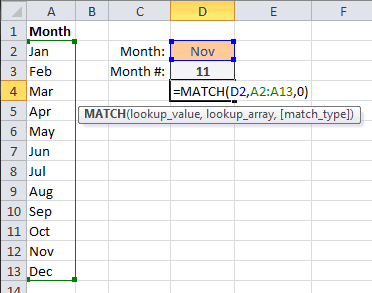The MATCH function in cell D3 returns 11, because that’s the position number of Nov in the range of month names.

I could go on, but you get the picture, right?

# Goal Seek in Excel

Formulas and Functions give you answers based upon input. If you know what a formula’s answer should be, but can’t derive the input value, Goal Seek is the tool for you.

I have a simple formula from my last post that takes one input, a Date in “m/d/yyyy” format, and returns the Day of the Year. This will tell me that Valentine’s Day — 14 Feb — is the 45th day of the year, or my birthday is day 311 this year.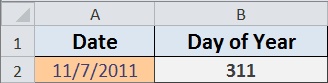The quickest way to find out what Date the 250th day of the year falls on is to use Goal Seek. For this example, here are the steps to take.

• Choose Data > What-If-Analysis > Goal Seek…
• In Excel for Mac and Excel 2003 choose Tools > Goal Seek…
• Click the Set cell box and select cell B2, which has the formula =A2-DATE(YEAR(A2),1,0)
• Click the To value box and type in 250, which is the outcome I want
• Click the By changing cell box and select cell A2, the value that Goal Seek will change
• Click OK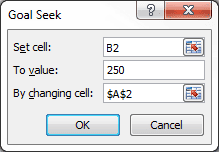Goal Seek searches for a solution and displays the following results.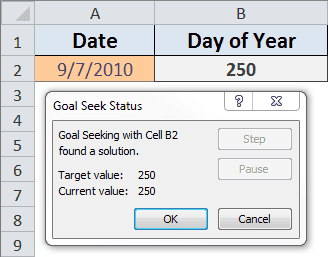Click OK to accept the changes, or click Cancel to restore the original value and leave the spreadsheet unchanged.

Note: Goal Seek works only with one variable input value.

Check out Goal Seek next time you know what a formula should be, but don’t have the time, inclination or smarts to figure it out.

# What Day of the Year is It?

Given the Date in this spreadsheet, a simple formula using the DATE Function will return the Day of Year. Go ahead and type a Date value in cell A2 to see how the formula works, the spreadsheet is embedded from my SkyDrive. (Date format is US, “m/d/yyyy”)

## The Day of Year Formula

The formula in cell B2 is:

= A2-DATE(YEAR(A2),1,0)

The DATE Function takes the Year from the Date you type in cell A2, and uses the zero (0) day of month 1 (January), which is the last day of the previous month. (I explained this in an earlier post).

The formula takes the Date you enter, and subtracts December 31st of the previous year to come up with the Day of Year.

You can copy the formula by double clicking cell B2, use Ctrl+A to select all the contents, and Ctrl+C to copy to the clipboard.

### [UPDATE] Problems with SkyDrive

Apparently there are some problems with using the embedded worksheet on this web page. I added a public link to the file on Windows SkyDrive, but that seems to have some issues, depending on whether you use a Mac or Windows computer.

If you have problems with the embedded worksheet on this webpage, or with the web version on SkyDrive let me know in the comments what problem(s) you’re having, what hardware (Windows computer, Mac computer, iOS device – iPad, or other device) and what web browser (Firefox, Chrome, Safari, Opera, IE) you’re using. Thanks so much.

# How Many Years, Months and Days Has it Been?

The DATEDIF Function comes in handy when you want to know how many years, months, and days there are between two dates. And even though it may be slightly flawed, it still beats the alternatives.

The following spreadsheet works well for birthdays or anniversaries. The DATEDIF Function is being used to generate the Years, Months, and Days.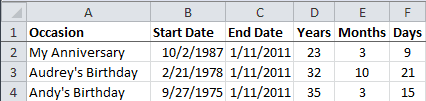The End Date column has the formula =TODAY().

The Years heading in cell D2 has the custom format ;;;”Years” which shows the text “Years” in the spreadsheet cell while hiding the underlying value “y” that you can see in the formula bar.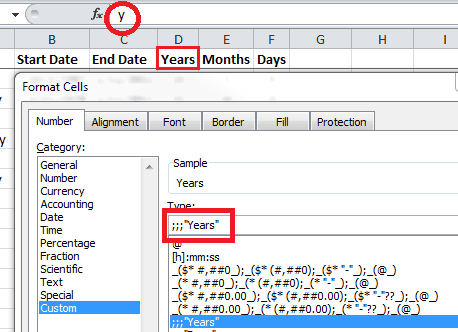The headings for Years, Months, and Days all have a custom format. The Underlying Value is what’s important for the next section.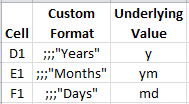## Using the DATEDIF Function

The DATEDIF function is present in all current versions of Excel, but is only documented in Excel 2000. It was provided for compatibility with Lotus 1-2-3.

The Syntax is:

DATEDIF(start_date, end_date, unit)

Start Date and End Date are self-explanatory, although the end date must come after start date. The Unit is the type of information you want to return.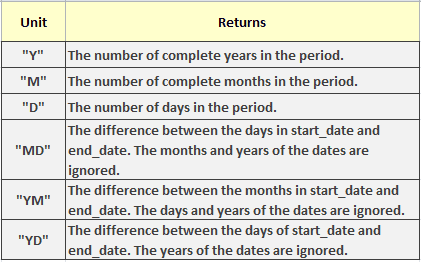The formula in cell D2 is =DATEDIF(\$B2,\$C2,D\$1) where \$B2 is the Start Date, \$C2 is the End Date, and D\$1 is the Unit. This gives you =DATEDIF(“10/2/1987”, “1/11/2011”, “y”) and returns 23 years.In the Months column I’m using the unit argument “ym” to return the number of months. (That’s the value in cell D1 you can’t see in the spreadsheet). The unit argument is “md” for the Days column.  The formulas in row 2 are:

• Years =DATEDIF(\$B2,\$C2,D\$1) = DATEDIF(“10/2/1987″,”1/11/2011″,”y”)
• Months =DATEDIF(\$B2,\$C2,E\$1) = DATEDIF(“2/21/1978″,”1/11/2011″,”ym”)
• Days =DATEDIF(\$B2,\$C2,F\$1) = DATEDIF(“9/27/1975″,”1/11/2011″,”md”)

## A Problem with DATEDIF Function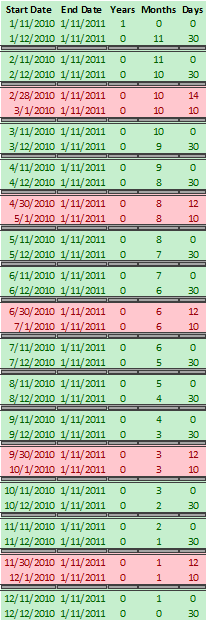I used a column of Start Dates for the past year and happened to notice a pattern with the Days column. Every month in the DATEDIF Function seems to have 31 days.

### The 31 Day Cycle

The first pair of dates are the 11th and 12th of 2010. The first date is 1 year, zero months and zero days from 1/11/2011. The second date is zero years, 11 months and 30 days from 1/11/2011.

The green pairs show the Days cycle from zero to 30 days, which means a 31 day cycle.

### Missing Days

Now look at the red pairs of data. These are the only non-sequential Days returned by the DATEDIF for the past year.

The first pair has start dates of 2/28/2010 and 3/1/2010. The first date is zero years, 10 months and 14 days from 1/11/2011. The second date is zero years, 10 months, and 10 days from 1/11/2011.

There are 3 days missing from this one day sequential difference.

The rest of the red pairs of data skip one day, which is the day after a month with less than 31 days.

For the spreadsheet I’ve put together this DATEDIF anomaly is not going to make me lose any sleep. Missing a day or two in 10 to 50 years is not very bothersome.

XLSX Format (Excel 2011, 2010, 2007, 2008)

XLS Format (Excel 2003, 2002, 2000)

# The TRIM Function in Excel and VBA

Excel’s TRIM Function is a great way to remove extra spaces before, after, and within text in a spreadsheet. However, if you think the identically named VBA function does the same thing you’d be sadly mistaken, as I was once upon a time.

Here’s a normal sentence.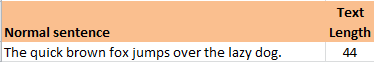Here’s a sentence with extra spaces – two before, one after, and several within the sentence.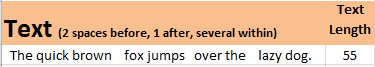Here’s a comparison of the two TRIM Functions.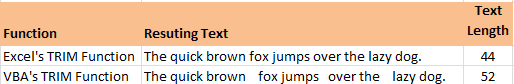The Excel TRIM function reemoves all spaces from text except for single spaces between words.

The VBA TRIM Function only removes leading and trailing spaces.

## The VBA TRIM Function Warning

If you need to remove spaces within text and are using VBA Trim function, it’s best to use Application.WorksheetFunction.Trim(your text here) to get the job done.

Note: This is a public service message. 🙂

# The VLOOKUP Function in Excel

VLOOKUP is an Excel Function that’s used to look up something. It takes four arguments. The first argument is the Something. The second and third arguments are the Look Up part. And the last argument is optional, but the most important, and is either TRUE or FALSE.

Technically the first argument is the lookup_value, but it’s just the Something we’re going to use in the Look Up part. The second and third arguments are the table that holds the look up data and column where the return value resides.

The VLOOKUP Function takes the Something and tries to find a match in the first column of the table.

Now here’s where the TRUE and FALSE part comes in. If the fourth argument is FALSE there will be an exact match. I use FALSE for virtually every VLOOKUP formula, so we’ll consider the TRUE option later.

So to continue, we have the Something lookup_value, and have found an exact match in the first column of the table. This also tells us the particular row of the table where our answer resides.

The third argument tells us the column number of the table to look in. Knowing the row and column gives us the cell where we can find the look up value.

## Using VLOOKUP with FALSE as the Last Argument

So a quick review. We use the VLOOKUP function, where the first argument is something like “Banana.” The second argument tells us where the table is that’s holding the lookup data. We match the word “Banana” in the first column of the table, then slide along the same row to the column number that’s given by the third argument and locate the cell holding the lookup value, which is then returned to the cell where we put the VLOOKUP formula. And of course all of this is guided by the fact that the last argument is FALSE, which tells the VLOOKUP function to get an exact match to “Banana.” (Upper and lower case are considered equivalent.)

Let’s look at a simple example. I have a worksheet named “MyData” that has a table with values we want to look up, shown below.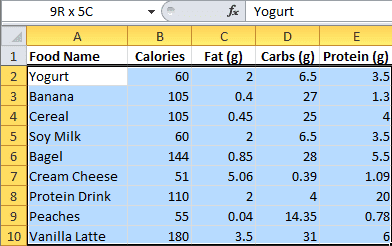Here’s a different worksheet with the VLOOKUP formula.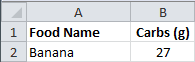Cell B2 holds the VLOOKUP formula:

=VLOOKUP(A2,MyData!\$A\$2:\$E\$10,4,FALSE)

The first argument is cell A2, which is “Banana.”  The second argument is the location of the data table on the MyData worksheet in the range A2:E10. The third argument is the number 4, because we want to look up Carbs (g) in the fourth column, and the last argument is FALSE to find an exact match.

Here’s the VLOOKUP dialog box that you can use in creating the formula. The picture rotates through the four different arguments — Lookup-Value, Table_array, Col_index_num, Range_lookup — so you can see the explanation for each.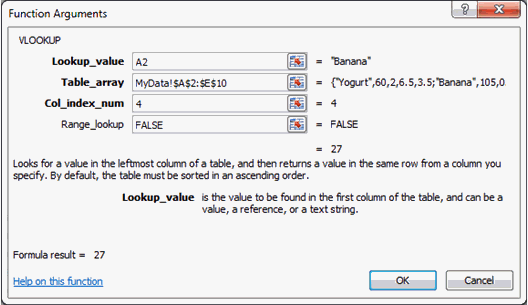One thing you need to know about using FALSE for the fourth argument is that, if there’s more than one of the Something, the VLOOKUP formula will only find the first one in the list.

## Using VLOOKUP with TRUE as the Last Argument

Here’s what you need to know about using TRUE as the last argument.

1. The values in the first column must be placed in ascending order, otherwise you might not get the correct value.
2. If you omit the last argument, VLOOKUP considers it to be TRUE.
3. With TRUE, you get an exact or approximate match. If no exact match is found, then you get the next largest value that’s less than the lookup_value. (And after all these years, this still strains my brain to near the breaking point.)

Here’s a simple example that shows how grades are returned by a VLOOKUP formula when given some numerical scores.  The data table for grades is shown here.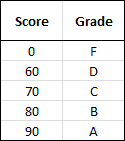• Any score with values from 0 – 59 will return an F grade.
• Any score with values 60 – 69 will return a D grade.
• Any score with values 70 – 79 will return a C grade.
• Any score with values 80 – 89 will return a B grade.
• Any score with values 90 – 100 will return an A grade.

I covered this in an earlier article: Grade Formulas in Excel.

Here’s the worksheet with Students, Scores, and the VLOOKUP formula.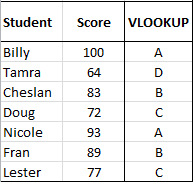And here’s the VLOOKUP formula used with TRUE as the fourth argument.

The first argument is the scores in the Score column, which I gave the name: Scores. The second argument is the table that I’ve named GradeLU. The third argument is 2 because the second column has the Grades.

If the first argument is 83, the VLOOKUP formula looks to the GradeLU table, and since the last argument is TRUE, it looks in the first column and matches the 80, then goes to the second column and returns the grade B.

# Logical Operations in Excel

Every once in a while you get fixated on something and the only way to let it go is to share it with others. So …. while reviewing the logical IF function and nested IF statements, I came up with a chart or table, that sort of evolved out of curiosity.

It started by looking at two conditions, both having true or false possibilities, as shown in the table below, where A and B have four possible outcomes.If you type the lowercase true or false into a cell they will change to uppercase TRUE or FALSE. That’s because Excel interprets them as logical functions.

You can also type =TRUE() or =FALSE() to get the same thing. Both functions have no arguments, yet return the logical values TRUE and FALSE, respectively.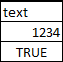Also take notice that text values align to the left side of a cell, numbers to the right, but logical values align to the middle.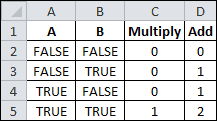TRUE and FALSE both have numerical values that you can see when mathematical operations are performed on them. In the table to the right, multiplying A times B gives values of zero (0) or one (1), but adding A and B gives values of zero (0), one (1), or two (2). Which proves that TRUE = 1 and FALSE = 0.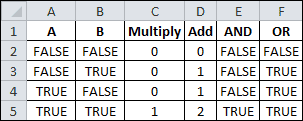When the outcomes for A and B are used as arguments for the AND and OR functions, it gives you a sense for the rules of how they operate.

The AND function will return TRUE if all of its logical arguments evaluate to TRUE. In this example, observation shows this to be the same as multiplying A and B together, given the numerical values of TRUE (1) and FALSE (0).

The OR function returns TRUE if any argument is TRUE, and returns FALSE if all arguments are FALSE.

Another logical function is NOT, which simply reverses the value of its logical TRUE or FALSE argument. Using AND inside the NOT function gives us the reverse of the AND function and is know as NAND. (see chart below)

Using the OR function inside the NOT function is called NOR and gives us the reverse of the OR function, as shown.Finally, using an equals (=) comparison between A and B is done to come up with a completely different set of TRUE and FALSE values for these four outcomes. It shows if A and B are the SAME.

Using the Equal comparison inside the NOT function gives us the reverse values, or not the same.

# Excel ISNUMBER or IsNumeric Function

I don’t like it when Excel worksheet functions are different from their VBA counterparts. Makes for some aggravation.

For example, I have a user generated data range that’s supposed to be made up of either numbers or empty cells. However, Excel users sometimes bump their keyboards inadvertently (when you hit the space bar) and things like a space character can get entered into an otherwise empty cell, and go undetected to the naked eye.

### VBA Programming

As part of a VBA macro I looped through each cell in this data range and performed an operation for any cell value greater than zero. This was a good plan for a data range having only number values or empty cells. (Well, not really as you’ll see below.)

However, I not only came across a space character in the user data, but also an accent character (`). As you can see in third column of the the chart pictured below, (>0) greater than zero is TRUE for the space character and the accent mark.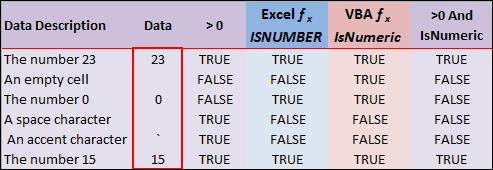What I really wanted was the Excel ISNUMBER fucntion that only shows TRUE when, well, there’s a number. (Fourth column)

So I tried the VBA Excel IsNumeric function in my macro, thinking it to be the same thing, and found that it didn’t work as I had imagined. For some reason the IsNumeric function shows TRUE for and empty cell as you can see in fifth column of the chart.

I wrote a function called IsNum() to simulate the VBA function IsNumeric in the worksheet.

```Function IsNum(data As Variant) If IsNumeric(data) Then IsNum = True Else IsNum = False End If End Function ```

The difference seems to be that the Worksheet Function ISNUMBER takes a Value for an argument, and the VBA function IsNumeric takes an Expression for its argument.

My solution is to use both greater than zero (>0) AND IsNumeric for my logic gate to perform further operations. (Last column in the chart) So in looping through the data range above, operations would only be performed on the numbers (23 and 15).

### Lessons Learned About Excel ISNUMBER or IsNumeric Function

1. Now I know that an accent character (`) is greater than zero.
2. The VBA function IsNumeric is different than the Excel worksheet function ISNUMBER.
3. Testing doesn’t always bring out all the ways a user can change the data. Only time and more users can do that.
4. One logical operation in a very large VBA macro (9 subroutines w/ 2 functions) can bring down the whole show.

# Calculate Hours Between Two Dates and Times in Excel

Recently I was asked how to subtract time in Excel (time difference) or how to calculate the number of hours between two points in time on different days. Since this was in a reader comment, I gave a brief answer that requires a fuller account here.

Dates and Times are all part of the master plan in Excel. Once you “get” the fundamentals, the rest is just icing on the cake.

A Date value in Excel looks like this: 40519
A Time value in Excel looks like this: 0.58333

Cell formatting changes how you see these numbers.

The Date: 7 Dec, 2010
The Time: 2:00 PM

## How to Calculate Time Between Dates in Excel or the Duration Between Two Dates

If you want to calculate time between dates in Excel or the duration between two dates, you need to understand what they mean first. When you type a Date into Excel, you may never see the underlying number, like 40519, but it’s there nonetheless. This a date serial number and it makes Date calculations easy.

You ask, “Why is this such a weird-looking number?” Well the Excel folks started a numbering system with Dates. In Excel for Windows they gave 1 Jan, 1900 the serial date number of 1, then continued numbering until this day and beyond. So serial number 40519 represents 7 Dec, 2010.

In Excel for Mac they started numbering Dates beginning with 2 Jan, 1904. (don’t ask) So the serial date 40519 represents 8 Dec, 2014 (Actually it’s known as the 1904 date system. To be clear, Macs can change Excel settings to use the 1900 date system).

## How to Subtract Time in Excel (Time Difference)

When you type 2:00 PM into a cell in Excel the underlying value is a fraction, but Excel interprets this as a time serial number and formats the cell accordingly.

Try typing 0.25 into a blank cell, then change the cell formatting to a TIME format, and you’ll get something like 6:00 AM.

As an aside, you can calculate this fraction for any time value during the day by taking the total number of seconds that have passed from midnight until your time value and dividing by 86,400 seconds in a day.## Dates and Times Together

In Excel the unit of time is “the Day,” a key fact to know. You’ll notice that Dates are integers, and Time is a fractional number. You can add the two together to get a Date/Time format.

So adding a Date serial number, like 40518, to a Time serial number, like 0.25, gives us 40518.25. Formatting the cell holding this value using “d mmm, yyyy h:mm AM/PM” will show 6 Dec, 2010 6:00 AM.

You can also enter something like 7 Dec, 2010 2:00 PM into a cell and Excel will recognize this as a Date/Time format. However, if you change the cell formatting to General, the underlying number is 40519.05833.

So hopefully by now you can see that subtracting two Date/Time formatted numbers can be done mathematically. Subtracting 6 Dec, 2010 6:00 AM from 7 Dec, 2010 2:00 PM is done by Excel “underneath the hood” as 40519.05833 – 40518.25 and the result is 1.3333.

## Calculating Hours Between 2 Dates and Times

If we recall that the unit of time is “the Day,” this value represents 1-1/3 days of time.  Since there are 24 hours in a day, converting to hours is a simple multiplication 24 * 1.3333 = or 32 hours. (24 * 4/3 to be more precise)

## Time Between Two Times / Dates

Finding the number of hours or the time between two times / dates is simple, just subtract the start date/time from the end date/time and multiply the result by 24 hours.

If you want to enter the dates and times separately (which is loads easier than typing in a date/time in one cell) then add the date/times together.

Hours = ((End_Date+End_Time)-(Start_Date+Start_Time))*24

Here’s a look at a typical worksheet designed to calculate the hours between two dates.As you can see, the formula for Hours, in cell F2, shows in the formula bar. And row 3 contains General formatting so you can view the date/time serial numbers for row 2.

Change the formatting for cells B2:E2 to match what you normally use for Date and Time data entry.

I hope this article helps you to better understand how to calculate time difference in Excel.